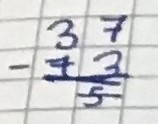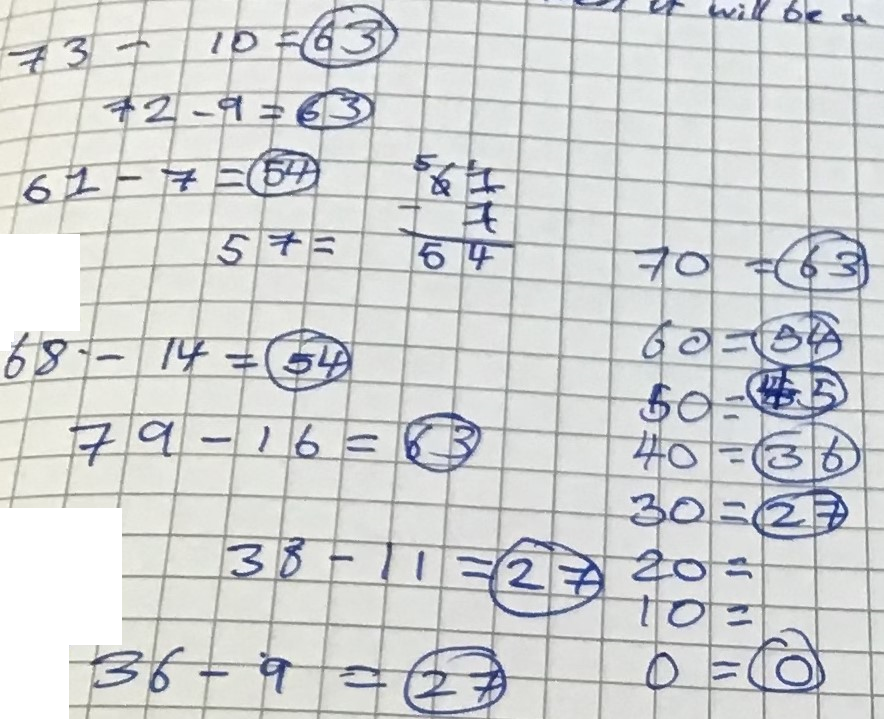#### You may also like### Days and Dates

Investigate how you can work out what day of the week your birthday will be on next year, and the year after...### Summing Consecutive Numbers

15 = 7 + 8 and 10 = 1 + 2 + 3 + 4. Can you say which numbers can be expressed as the sum of two or more consecutive integers?### Always the Same

Arrange the numbers 1 to 16 into a 4 by 4 array. Choose a number. Cross out the numbers on the same row and column. Repeat this process. Add up you four numbers. Why do they always add up to 34?

# Always a Multiple?

##### Age 11 to 14 Challenge Level:

Take any two-digit number. Reverse the digits, and subtract your answer from your original number. What do you notice?
Evie and Rose from Channing in the UK sent in an observation:You notice that if the second digit is higher than the first, the total
will be a minus number and the opposite effect if the second number is
smaller.

Archisman from The Future Foundation School in India, Leopold from Kings School Al Barsha in UAE and Shriya from International School Frankfurt in Germany noticed something else. They explained it using Charlie's method. This is Shriya's work:
e.g. $72-27=45$
$45$ is a multiple of $9$.
e.g. $97-79=18$
$18$ is also a multiple of $9.$
This is why:
$(10a+b) -10b -a= 9a-9b$

Take any two-digit number. Add its digits, and subtract your answer from your original number. What do you notice?
Evie and Rose noticed that:You notice that all the ‘tens’ have their own answer, and when the totals of each ten are put in order, they go up in nines. For example any number from 70 to 79 when put through the number trick, total up to 63, numbers from 80 to 89 total to 72 etc.

Monel and Rasel, from Globe Academy, explained why:
If you have $36$ (for example) and you take away the units digit first, then you will always get a multiple of $10.$
If you then take away the tens digit you will get a multiple of $9$ (because $3\times10 - 3\times1 = 3\times9).$

Leopold, Shriya and Archisman all explained this using Charlie's method. This is Archisman's work:
Let the number be $10x+y$
Sum of digits $=x+y$
Original number $-$ Sum of digits $=10x+y-(x+y)$
$=10x+y-x-y$
$=9x$
Hence,we observe that the result is a multiple of $9$ or to be more specific $9$ multiplied by the tens digit of the number.
Example: $92-11=81$ which is $9$ multiplied by $9$ (the tens digit of the number)

Take any three-digit/five-digit number. Reverse the digits, and subtract your answer from your original number. What do you notice?
Leopold, Archisman and Shriya investigated these questions using Charlie's method. Here is Leopold's work:

HUNDRED TEN UNIT
$A$      $B$           $C$
$C$ $B$ $A$
$100A+10B+C\\ 100C+10B+A\\ (100A+10B+C)-(100C+10B+A)\\ =99A-99C\\ =99(A-C)\\ =11(9A-9C)\\ =9(11A-11C)$
Thus it will always lead to a multiple of $99,11$ and $9.$

Five digits:
 TEN THOUSAND THOUSAND HUNDRED TEN UNIT $A$ $B$ $C$ $D$ $E$ $E$ $D$ $C$ $B$ $A$
$10000A+1000B+100C+10D+E\\ 10000E+1000D+100C+10B+A\\ (10000A+1000B+100C+10D+E)-(10000E+1000D+100C+10B+A)\\ =99,999A+990B-990D-99,999E\\ =9(11,111A+110B-110D-11,111E)$
Thus it will alway be a multiple of nine

Archisman and Shriya found more factors in the five digit case. This is Shriya's work:
$(10000a+1000b+100c+10d+e) -10000e -1000d -100c -10b -a\\= 9999a+990b-990d-9999e$
$9999$ and $990$ are multiples of $9$ and $11.$

And Archisman concluded:
$9999x+990y-990a-9999b =99(101x+10y-10a-101b)$
Hence,we observe that the result is a multiple of $9,11$ and $99$.
Example: $54321-12345=41976$ which is a multiple of $9,11,99$